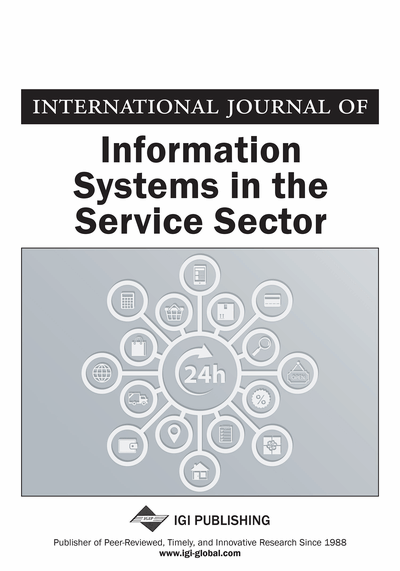# Towards a Faster Image Segmentation Using the K-means Algorithm on Grayscale Histogram

Lamine Benrais (LRIA Laboratory, Computer Science Department, University of Science and Technology Houari Boumediene, Algiers, Algeria) and Nadia Baha (LRIA Laboratory, Computer Science Department, University of Science and Technology Houari Boumediene, Algiers, Algeria)
DOI: 10.4018/IJISSS.2016040105
Available
\$37.50
No Current Special Offers

## Abstract

The K-means is a popular clustering algorithm known for its simplicity and efficiency. However the elapsed computation time is one of its main weaknesses. In this paper, the authors use the K-means algorithm to segment grayscale images. Their aim is to reduce the computation time elapsed in the K-means algorithm by using a grayscale histogram without loss of accuracy in calculating the clusters centers. The main idea consists of calculating the histogram of the original image, applying the K-means on the histogram until the equilibrium state is reached, and computing the clusters centers then the authors use the clusters centers to run the K-means for a single iteration. Tests of accuracy and computational time are presented to show the advantages and inconveniences of the proposed method.
Article Preview
Top

Many research efforts have been made to improve the K-means computation time and its convergence. Similar approaches to our proposed method have been done using the HSV color space (Tse-Wei et al., 2008; Zadeh, 2013) for grayscale and colored image, and a very fast computation time has been noticed with a good clustering efficiency. Another of the many approaches treats the change in the distance metric used between intra-clusters. The Euclidean (Danielsson & Per-Erik, 1980) distance is the more often used as in (Wang et al., 2005), the result of the clusters is spherical or ball-shaped and usually used for data in two or three dimension (Anil, 2010; Su et al., 2001) and also gives good results when the clusters are compact or outlying (Anil et al., 1999). More variations on how to calculate the distances have been developed, such as the Minkowski distance metric (Ridder, 1992; Ichino et al., 1994), which is a more general formula than the Euclidean distance metric such in (Archana, S et al., 2013; Anil, 2010; De Amorim et al., 2012). The Manhattan distance (Pieterse & Paul, 2006), a more specific formula than the Euclidian distance, can also be used as a distance metric as in (Archana, et al., 2013; Anil, 2010; Kahkashan & Sunita, 2013). The Mahalanobis distance metric (De Maesschalck et al., 2000) is also used in (Xiang et al., 2008) for data with high dimensional size. The Itakura–Saito distance (Enqvist & Karlsson, 2008) is also used in vector quantization for speech processing (Anil, 2010). All distances metrics aim to reduce the computation time of the K-means algorithm and try to make the algorithm converge faster.

## Complete Article List

Search this Journal:
Reset
Open Access Articles
Volume 14: 4 Issues (2022): 2 Released, 2 Forthcoming
Volume 13: 4 Issues (2021)
Volume 12: 4 Issues (2020)
Volume 11: 4 Issues (2019)
Volume 10: 4 Issues (2018)
Volume 9: 4 Issues (2017)
Volume 8: 4 Issues (2016)
Volume 7: 4 Issues (2015)
Volume 6: 4 Issues (2014)
Volume 5: 4 Issues (2013)
Volume 4: 4 Issues (2012)
Volume 3: 4 Issues (2011)
Volume 2: 4 Issues (2010)
Volume 1: 4 Issues (2009)
View Complete Journal Contents Listing